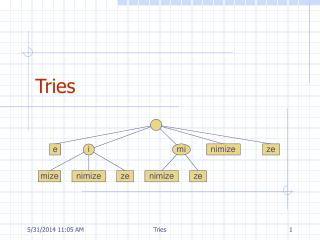DownloadDownload PresentationTries

# Tries

Download Presentation## Tries

- - - - - - - - - - - - - - - - - - - - - - - - - - - E N D - - - - - - - - - - - - - - - - - - - - - - - - - - -
##### Presentation Transcript

1. Tries Tries

2. Outline and Reading • Standard tries (§9.2.1) • Compressed tries (§9.2.2) • Suffix tries (§9.2.3) • Huffman encoding tries (§9.3.1) Tries

3. Preprocessing the pattern speeds up pattern matching queries After preprocessing the pattern, KMP’s algorithm performs pattern matching in time proportional to the text size If the text is large, immutable and searched for often (e.g., works by Shakespeare), we may want to preprocess the text instead of the pattern A trie is a compact data structure for representing a set of strings, such as all the words in a text A tries supports pattern matching queries in time proportional to the pattern size Preprocessing Strings Tries

4. Standard Trie (1) • The standard trie for a set of strings S is an ordered tree such that: • Each node but the root is labeled with a character • The children of a node are alphabetically ordered • Each external node is associated with a string in s, such that the concatenation of the labels of the nodes on the path from the root to an external node v yields the string associated with v. • Example: standard trie for the set of strings S = { bear, bell, bid, bull, buy, sell, stock, stop } Tries

5. Standard Trie (2) • A standard trie uses O(n) space and supports searches, insertions and deletions in time O(dm), where: n total size of the strings in S m size of the string parameter of the operation d size of the alphabet Tries

6. Word Matching with a Trie • We insert the words of the text into a trie • Each leaf stores the occurrences of the associated word in the text Tries

7. Compressed Trie • A compressed trie has internal nodes of degree at least two • It is obtained from standard trie by compressing chains of “redundant” nodes • An internal node is redundant if it has only one child Tries

8. Compact Representation • Compact representation of a compressed trie for an array ofstrings: • Stores at the nodes ranges of indices instead of substrings • Uses O(s) space, where s is the number of strings in the array • Serves as an auxiliary index structure Tries

9. Suffix Trie (1) • The suffix trie of a string X is the trie of all the suffixes of X Tries

10. Suffix Trie (2) • Compact representation of the suffix trie for a string X of size n from an alphabet of size d • Uses O(n) space • Supports arbitrary pattern matching queries in X in O(dm) time, where m is the size of the pattern Tries

11. Text Compression • Given a string X defined over a alphabet, to efficiently encode X into a small binary string. • Low-bandwidth channel • Large documents Tries

12. a d e b c Encoding Trie (1) • A code is a mapping of each character of an alphabet to a binary code-word • A prefix code is a binary code such that no code-word is the prefix of another code-word • An encoding trie represents a prefix code • Each leaf stores a character • The code-word of a character is given by the path from the root to the leaf storing the character (0 for a left child and 1 for a right child Tries

13. c a d b b r a c r d Encoding Trie (2) • Given a text string X, we want to find a prefix code for the characters of X that yields a small encoding for X • Frequent characters should have short code-words • Rare characters should have long code-words • Example • X =abracadabra • T1 encodes X into 29 bits • T2 encodes X into 24 bits T1 T2 Tries

14. Huffman’s Algorithm AlgorithmHuffmanEncoding(X) Inputstring X of size n Outputoptimal encoding trie for X C distinctCharacters(X) computeFrequencies(C, X) Qnew empty heap for all c  C Tnew single-node tree storing c Q.insert(getFrequency(c), T) while Q.size()> 1 f1 Q.minKey() T1 Q.removeMin() f2 Q.minKey() T2 Q.removeMin() Tjoin(T1, T2) Q.insert(f1+ f2, T) return Q.removeMin() • Given a string X, Huffman’s algorithm construct a prefix code that minimizes the size of the encoding of X • It runs in timeO(n + d log d), where n is the size of X and d is the number of distinct characters of X • A heap-based priority queue is used as an auxiliary structure Tries

15. 2 4 a c d b r 5 6 a b c d r 2 4 5 2 1 1 2 11 a c d b r 6 a 5 2 2 4 a b c d r c d b r 5 2 2 Example X= abracadabra Frequencies Tries